library(tidyverse)
## ── Attaching packages ────────────────────────────────────────── tidyverse 1.2.1 ──
## ✔ ggplot2 3.3.2     ✔ purrr   0.3.3
## ✔ tibble  2.1.3     ✔ dplyr   0.8.3
## ✔ tidyr   1.0.0     ✔ stringr 1.4.0
## ✔ readr   1.3.1     ✔ forcats 0.4.0
## Warning: package 'ggplot2' was built under R version 3.6.2
## ── Conflicts ───────────────────────────────────────────── tidyverse_conflicts() ──
## ✖ dplyr::lag()    masks stats::lag()
library(knitr)

options(scipen = 4)

### Final project practice: Analysis of a different NLSY data set

For the purpose of this analysis we’re going to look at a different version of the NLSY data set. Here I’m loading in data from the 1979 cohort. Note that for your final project you’re working with a more contemporary cohort, the 1997 cohort.

nlsy <- read_csv("http://www.andrew.cmu.edu/user/achoulde/94842/final_project/nlsy79/nlsy79_income.csv")
## Parsed with column specification:
## cols(
##   .default = col_double()
## )
## See spec(...) for full column specifications.
# Change column names (mostly) to question name abbreviations
colnames(nlsy) <- c("VERSION_R25_2012",
"CASEID_1979",
"FAM-2A_1979",
"FAM-POB_1979",
"FAM-3_1979",
"FAM-3A_1979",
"FAM-RES_1979",
"FAM-6_1979",
"R_REL-1_COL_1979",
"SCHOOL-31_1979",
"MIL-6_1979",
"WOMENS-ROLES_000001_1979",
"WOMENS-ROLES_000002_1979",
"WOMENS-ROLES_000003_1979",
"WOMENS-ROLES_000004_1979",
"WOMENS-ROLES_000006_1979",
"WOMENS-ROLES_000007_1979",
"WOMENS-ROLES_000008_1979",
"EXP-OCC_1979",
"EXP-9_1979",
"race",
"gender",
"MARSTAT-KEY_1979",
"FAMSIZE_1979",
"POVSTATUS_1979",
"POLICE-1_1980",
"POLIC-1C_1980",
"POLICE-2_1980",
"ALCH-2_1983",
"DS-8_1984",
"DS-9_1984",
"Q13-5_TRUNC_REVISED_1990",
"POVSTATUS_1990",
"HGCREV90_1990",
"jobs.num",
"NUMCH90_1990",
"AGEYCH90_1990",
"DS-12_1998",
"DS-13_1998",
"INDALL-EMP.01_2000",
"CPSOCC80.01_2000",
"OCCSP-55I_CODE_2000",
"Q2-15B_2000",
"Q10-2_2000",
"Q13-5_TRUNC_REVISED_2000",
"FAMSIZE_2000",
"TNFI_TRUNC_2000",
"POVSTATUS_2000",
"MARSTAT-COL_2000",
"MARSTAT-KEY_2000",
"MO1M1B_XRND",
"Q2-10B~Y_2012",
"INDALL-EMP.01_2012",
"OCCALL-EMP.01_2012",
"OCCSP-55I_CODE_2012",
"Q2-15A_2012",
"Q12-6_2012",
"income",
"Q13-5_SR000001_2012",
"Q13-5_SR000002_2012",
"Q13-18_TRUNC_2012",
"Q13-18_SR000001_TRUNC_2012",
"FAMSIZE_2012",
"REGION_2012",
"HGC_2012",
"URBAN-RURAL_2012",
"JOBSNUM_2012")

# Map all negative values to missing (NA)
# DO NOT DO THIS IN YOUR PROJECT WITHOUT CAREFUL JUSTIFICATION
nlsy[nlsy < 0] <- NA

We’ll now walk through the following tasks: - Running a regression model - Using anova() to compare two nested regression models to assess whether a categorical variable is statistically significant - Updating models to include interaction terms - Interpreting coefficients of categorical x categorical interaction terms - Testing for statistial significance of an interaction term in a model - Getting a similar but simplified analysis with figures and summary tables

#### (a) Run a linear regression modeling income as a linear function of gender, race and jobs.num.

# Transform and relabel gender and race variables
nlsy <- mutate(nlsy,
gender = recode_factor(gender,
2 = "Female",
1 = "Male"),
race = recode_factor(race,
2 = "Black",
3 = "Other",
1 = "Hispanic"))

Here we’re setting “Female” as the baseline category for the gender variable and “Black” as the baseline for the race category. For race, “Other” (non-Black, non-Hispanic, a category that includes “White”) would be a more natural baseline for interpretability purposes. In your final project you’ll want to think carefully about what baseline categories you select. I am not picking the best baselines here because I want to show you how to interpret coefficients in general, even if the baseline isn’t ideally chosen.

# Run regression
nlsy.lm <- lm(income ~ gender + race + jobs.num, data = nlsy)

# Output summary
summary(nlsy.lm)
##
## Call:
## lm(formula = income ~ gender + race + jobs.num, data = nlsy)
##
## Residuals:
##    Min     1Q Median     3Q    Max
## -66845 -29250 -11189  14642 319529
##
## Coefficients:
##              Estimate Std. Error t value   Pr(>|t|)
## (Intercept)   19079.7     1632.6  11.686    < 2e-16 ***
## genderMale    24895.5     1316.5  18.910    < 2e-16 ***
## raceOther     22870.1     1507.0  15.176    < 2e-16 ***
## raceHispanic   8929.8     1899.9   4.700 0.00000265 ***
## jobs.num       -370.8      146.4  -2.533     0.0113 *
## ---
## Signif. codes:  0 '***' 0.001 '**' 0.01 '*' 0.05 '.' 0.1 ' ' 1
##
## Residual standard error: 53620 on 6738 degrees of freedom
##   (5943 observations deleted due to missingness)
## Multiple R-squared:  0.08067,    Adjusted R-squared:  0.08012
## F-statistic: 147.8 on 4 and 6738 DF,  p-value: < 2.2e-16

#### (b) Use the anova() function to assess whether race is a statistically significant predictor of income by comparing your model from part (a) to a model with just gender and jobs.num.

Hint: You can use the update() function to drop predictors from a model.

anova(update(nlsy.lm, . ~ . - race), nlsy.lm)
## Analysis of Variance Table
##
## Model 1: income ~ gender + jobs.num
## Model 2: income ~ gender + race + jobs.num
##   Res.Df            RSS Df    Sum of Sq      F    Pr(>F)
## 1   6740 20059555089013
## 2   6738 19369215650204  2 690339438809 120.07 < 2.2e-16 ***
## ---
## Signif. codes:  0 '***' 0.001 '**' 0.01 '*' 0.05 '.' 0.1 ' ' 1

Race turns out to be a highly statistically signficant predictor of income in the model. You can see this also by looking at the coefficient estimates and standard errors — the estimated coefficients are very large, with relatively small standard errors.

#### (c) Update your linear regression model from part (a) to also include an interaction term between race and gender.

nlsy.lm.interact <- update(nlsy.lm, . ~ . + race*gender)

summary(nlsy.lm.interact)
##
## Call:
## lm(formula = income ~ gender + race + jobs.num + gender:race,
##     data = nlsy)
##
## Residuals:
##    Min     1Q Median     3Q    Max
## -71941 -29710 -10500  14716 316605
##
## Coefficients:
##                         Estimate Std. Error t value   Pr(>|t|)
## (Intercept)              25837.0     1859.0  13.898    < 2e-16 ***
## genderMale                9551.9     2361.5   4.045 0.00005293 ***
## raceOther                 9924.3     2084.9   4.760 0.00000198 ***
## raceHispanic              4149.5     2620.5   1.583     0.1134
## jobs.num                  -276.2      146.0  -1.892     0.0585 .
## genderMale:raceOther     26627.8     2985.7   8.918    < 2e-16 ***
## genderMale:raceHispanic   9788.9     3778.6   2.591     0.0096 **
## ---
## Signif. codes:  0 '***' 0.001 '**' 0.01 '*' 0.05 '.' 0.1 ' ' 1
##
## Residual standard error: 53290 on 6736 degrees of freedom
##   (5943 observations deleted due to missingness)
## Multiple R-squared:  0.09194,    Adjusted R-squared:  0.09113
## F-statistic: 113.7 on 6 and 6736 DF,  p-value: < 2.2e-16

#### (d) How many coefficients are estimated for this interaction term?

There are 2 estimated coefficients.

#### (e) What do you think these coefficients mean? How would you interpret them?

coef.race.other <- round(coef(nlsy.lm.interact)["genderMale:raceOther"], 0)

coef.race.other
## genderMale:raceOther
##                26628

#### Testing significance of the interaction term with anova

By looking at the individual p-values for the interaction term coefficients, we can answer the question of whether the difference in income gap differs between Black individuals and those of a different given race category. To more directly answer the question of whether the observed differences in income gap across the race categories are statistically significant, we need to use the anova command.

anova(nlsy.lm, nlsy.lm.interact)
## Analysis of Variance Table
##
## Model 1: income ~ gender + race + jobs.num
## Model 2: income ~ gender + race + jobs.num + gender:race
##   Res.Df            RSS Df    Sum of Sq      F    Pr(>F)
## 1   6738 19369215650204
## 2   6736 19131704011553  2 237511638651 41.812 < 2.2e-16 ***
## ---
## Signif. codes:  0 '***' 0.001 '**' 0.01 '*' 0.05 '.' 0.1 ' ' 1

The p-value is statistically significant, so we can reject the null hypothesis that the income gap is the same across all the race categories. In other words, the data suggests that the income gap between men and women does vary with race.

#### The regression-free approach

In the previous examples we used a regression because we wanted to adjust for the jobs.num variable. However, before delving into a regression, you can get a lot of the same insights with some simple plots and tables.

Here’s a set of commands that calculates the difference in average income between men and women for each race category.

nlsy %>%
group_by(race) %>%
summarize(income.gap = mean(income[gender == "Male"], na.rm = TRUE) -
mean(income[gender == "Female"], na.rm = TRUE))
## # A tibble: 3 x 2
##   race     income.gap
##   <fct>         <dbl>
## 1 Black         8683.
## 2 Other        35543.
## 3 Hispanic     18190.

This is a great, easy-to-interpret table that you can include in your analysis. (Remember to round! — not shown here.) By changing out race for other categorical variables, you can try to identify other factors that may affect the income gap.

Here’s a one-line plotting command that summarizes the previous table in an east-to-interpret plot.

gap.data <- nlsy %>%
group_by(race) %>%
summarize(income.gap = mean(income[gender == "Male"], na.rm = TRUE) -
mean(income[gender == "Female"], na.rm = TRUE))

ggplot(data = gap.data, aes(x = race, y = income.gap, fill = race)) +
geom_bar(stat = "identity") +
xlab("Race") +
ylab("Income gap($)") + ggtitle("Income gap between men and women, by race") + guides(fill = FALSE)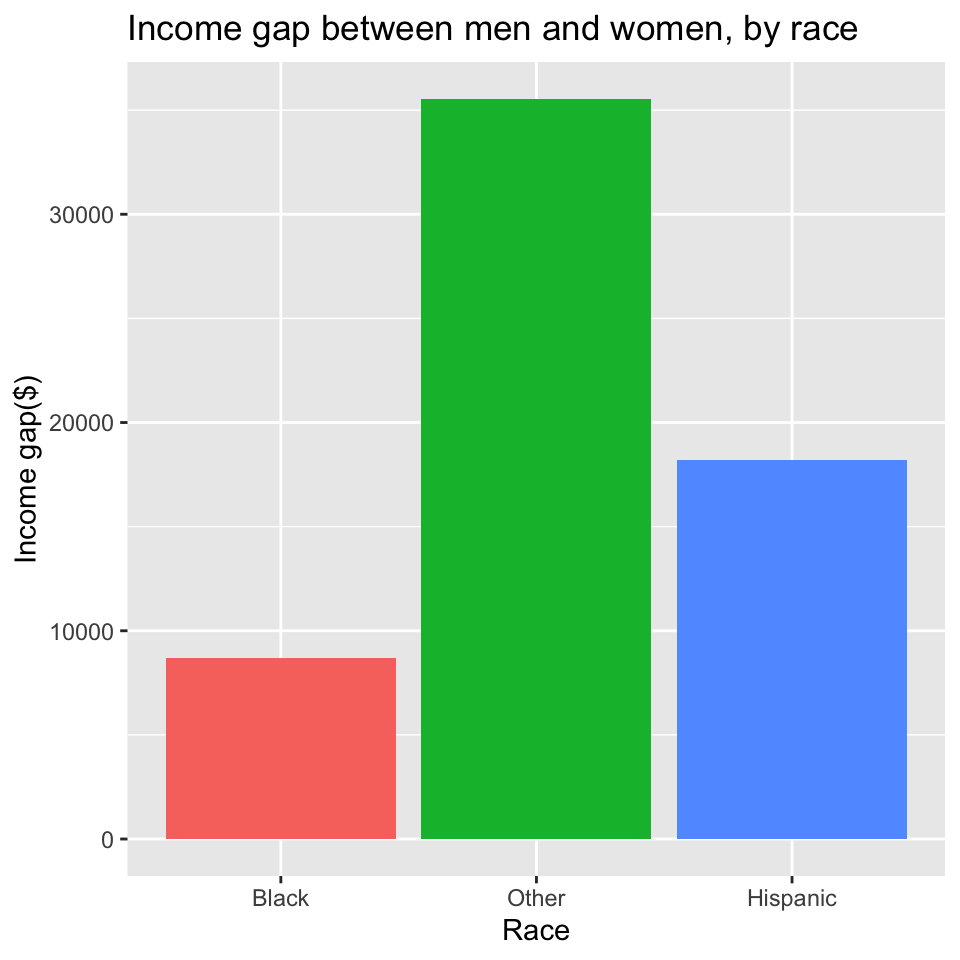We’ll now look at a couple of ways of modifying/improving this graphic. 1. Re-order the variables on the x axis so that the bars are sorted by in descending order of height. i.e., plot the bars in descending order of wage gap. 2. By running t-tests you can obtain confidence intervals for the income gaps. You can plot these using the geom_errorbar layer in ggplot. #### Improving the figure ##### Step 1: Reordering the bars This is a simple matter of reordering the race variable in the gap.data data frame according to the value of income.gap. The plotting command itself remains unchanged. # Reorder race factor levels according to -income.gap # this is the same as reordering in *descending* order of # income gap gap.data <- mutate(gap.data, race = reorder(race, -income.gap)) # Same plotting command as before ggplot(data = gap.data, aes(x = race, y = income.gap, fill = race)) + geom_bar(stat = "identity") + xlab("Race") + ylab("Income gap($)") +
ggtitle("Income gap between men and women, by race") +
guides(fill = FALSE)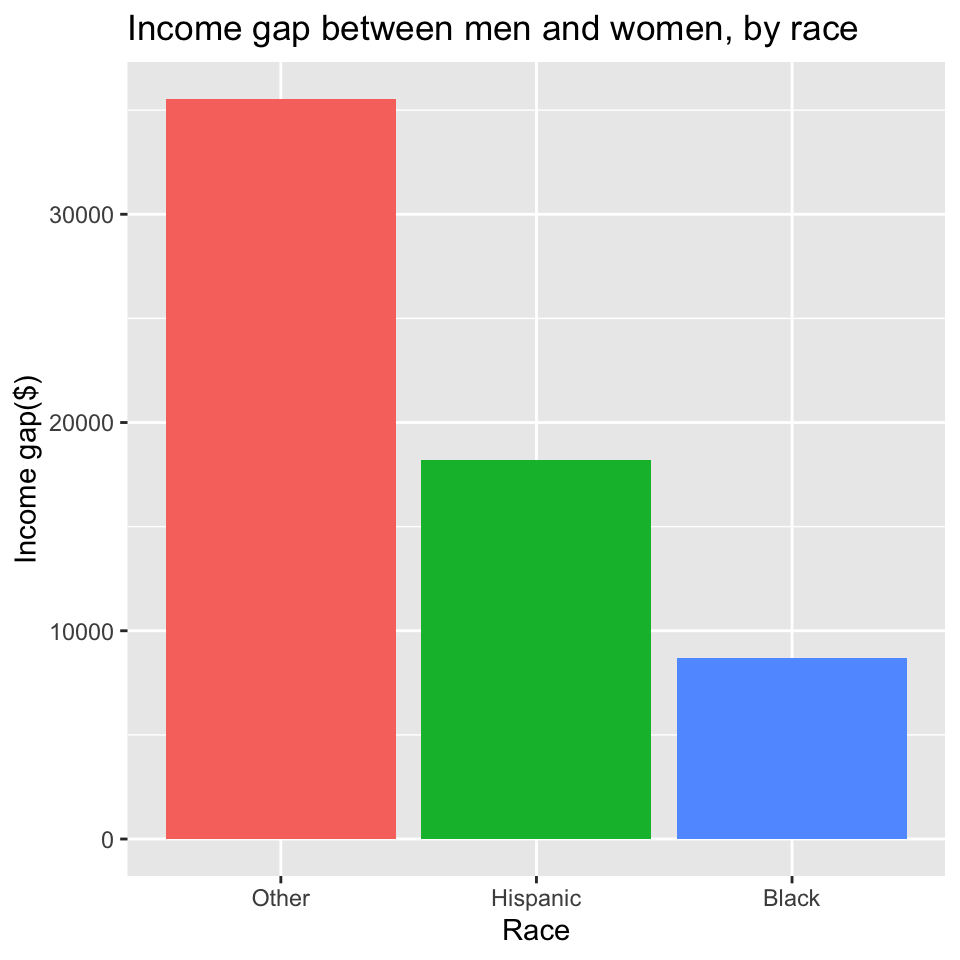Note: This changes which bar color gets used for which race. If race is used several times in your analysis, you probably don’t want the mapping between colors and race to keep changing. To address this, you may want to manually specify the color mapping, or just use a single color for all the bars in the above plot. E.g.,

ggplot(data = gap.data, aes(x = race, y = income.gap)) +
geom_bar(stat = "identity", fill = I('steelblue')) +
xlab("Race") +
ylab("Income gap($)") + ggtitle("Income gap between men and women, by race") + guides(fill = FALSE)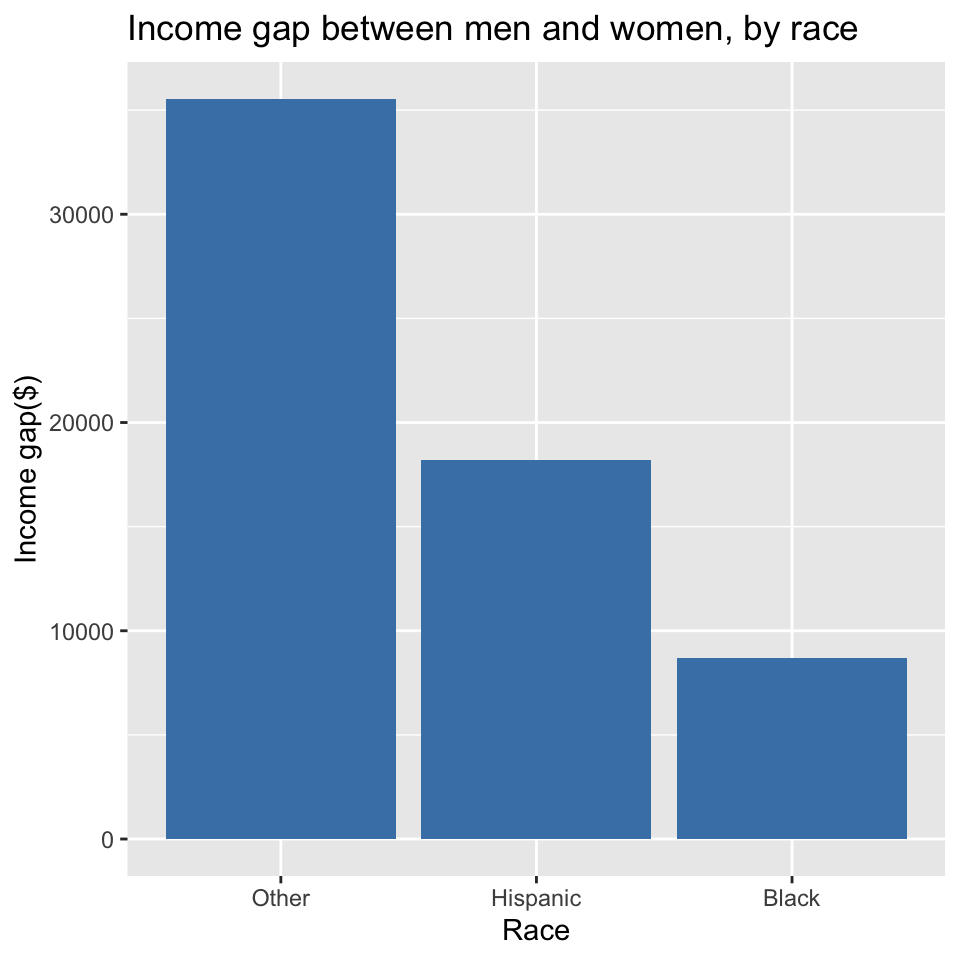##### Step 2: Adding error bars using t-test confidence intervals Now that we’ve introduced the plyr package, we can quite easily put together all the information we need in order to add error bars to our plot. First, note that when we run a t.test with formula income ~ gender the difference that’s calculated is female income - male income. We want male income - female income. Thus when we get a confidence interval from the t-test, we want to flip it and change the sign. i.e., if [-8000, -2000] is a 95% confidence interval for $$\mu_F - \mu_M$$, then [2000, 8000] is a 95% confidence interval for $$\mu_M - \mu_F$$. This is what the code below does to calculate error bars for our plot. # Calculate income gaps (male - female) and 95% confidence intervals # for the gap gap.data.conf <- nlsy %>% group_by(race) %>% summarize(income.gap = mean(income[gender == "Male"], na.rm = TRUE) - mean(income[gender == "Female"], na.rm = TRUE), upper = -t.test(income ~ gender)$conf.int,
lower = -t.test(income ~ gender)$conf.int, is.significant = as.numeric(t.test(income ~ gender)$p.value < 0.05))

# Re-order the race factor according to gap size
gap.data.conf <- mutate(gap.data.conf,
race = reorder(race, income.gap))

# Plot, with error bars
ggplot(data = gap.data.conf, aes(x = race, y = income.gap,
fill = is.significant)) +
geom_bar(stat = "identity") +
xlab("Race") +
ylab("Income gap(\$)") +
ggtitle("Income gap between men and women, by race") +
guides(fill = FALSE) +
geom_errorbar(aes(ymax = upper, ymin = lower), width = 0.1, size = 1) +
theme(text = element_text(size=12))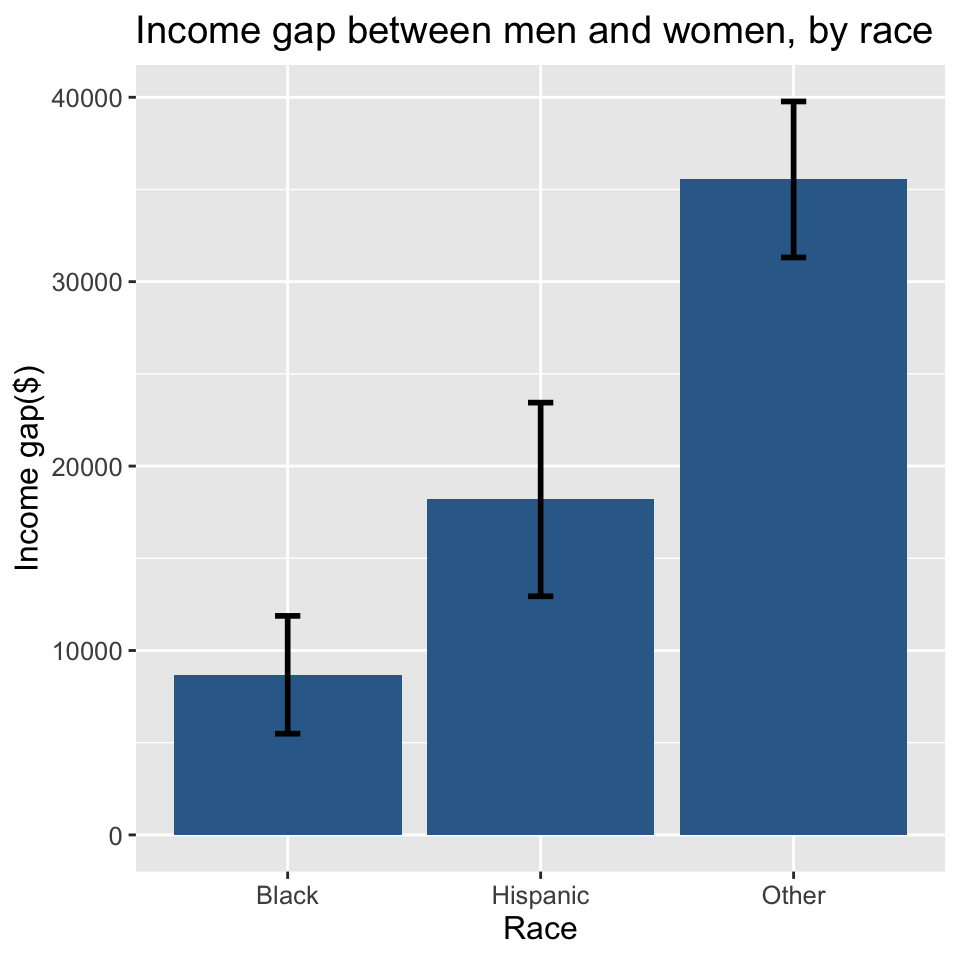## Stratified regressions with ggplot and dplyr

#### Gapminder life expectancy data

gapminder <- read_delim("http://www.andrew.cmu.edu/user/achoulde/94842/data/gapminder_five_year.txt",
delim = "\t") # Load data
## Parsed with column specification:
## cols(
##   country = col_character(),
##   year = col_double(),
##   pop = col_double(),
##   continent = col_character(),
##   lifeExp = col_double(),
##   gdpPercap = col_double()
## )
gapminder # A look at the data
## # A tibble: 1,704 x 6
##    country      year      pop continent lifeExp gdpPercap
##    <chr>       <dbl>    <dbl> <chr>       <dbl>     <dbl>
##  1 Afghanistan  1952  8425333 Asia         28.8      779.
##  2 Afghanistan  1957  9240934 Asia         30.3      821.
##  3 Afghanistan  1962 10267083 Asia         32.0      853.
##  4 Afghanistan  1967 11537966 Asia         34.0      836.
##  5 Afghanistan  1972 13079460 Asia         36.1      740.
##  6 Afghanistan  1977 14880372 Asia         38.4      786.
##  7 Afghanistan  1982 12881816 Asia         39.9      978.
##  8 Afghanistan  1987 13867957 Asia         40.8      852.
##  9 Afghanistan  1992 16317921 Asia         41.7      649.
## 10 Afghanistan  1997 22227415 Asia         41.8      635.
## # … with 1,694 more rows

#### Example: Fitting a linear model for each country

We’re now going to go through an example where we get the life expectancy in 1952 and the rate of change in life expectancy over time for each country. The rate of change will be obtained by regressing lifeExp on year.

##### (1) Figure out how to extract the desired information.

country.name <- "Ireland"  # Pick a country
gapminder.sub <- filter(gapminder, country == country.name)  # Pull data for this country
gapminder.sub
## # A tibble: 12 x 6
##    country  year     pop continent lifeExp gdpPercap
##    <chr>   <dbl>   <dbl> <chr>       <dbl>     <dbl>
##  1 Ireland  1952 2952156 Europe       66.9     5210.
##  2 Ireland  1957 2878220 Europe       68.9     5599.
##  3 Ireland  1962 2830000 Europe       70.3     6632.
##  4 Ireland  1967 2900100 Europe       71.1     7656.
##  5 Ireland  1972 3024400 Europe       71.3     9531.
##  6 Ireland  1977 3271900 Europe       72.0    11151.
##  7 Ireland  1982 3480000 Europe       73.1    12618.
##  8 Ireland  1987 3539900 Europe       74.4    13873.
##  9 Ireland  1992 3557761 Europe       75.5    17559.
## 10 Ireland  1997 3667233 Europe       76.1    24522.
## 11 Ireland  2002 3879155 Europe       77.8    34077.
## 12 Ireland  2007 4109086 Europe       78.9    40676.
# Scatterplot of life exp vs year
# with a regression line overlaid
qplot(year, lifeExp, data = gapminder.sub, main = paste("Life expectancy in", country.name)) +
geom_smooth(method = "lm")
## geom_smooth() using formula 'y ~ x'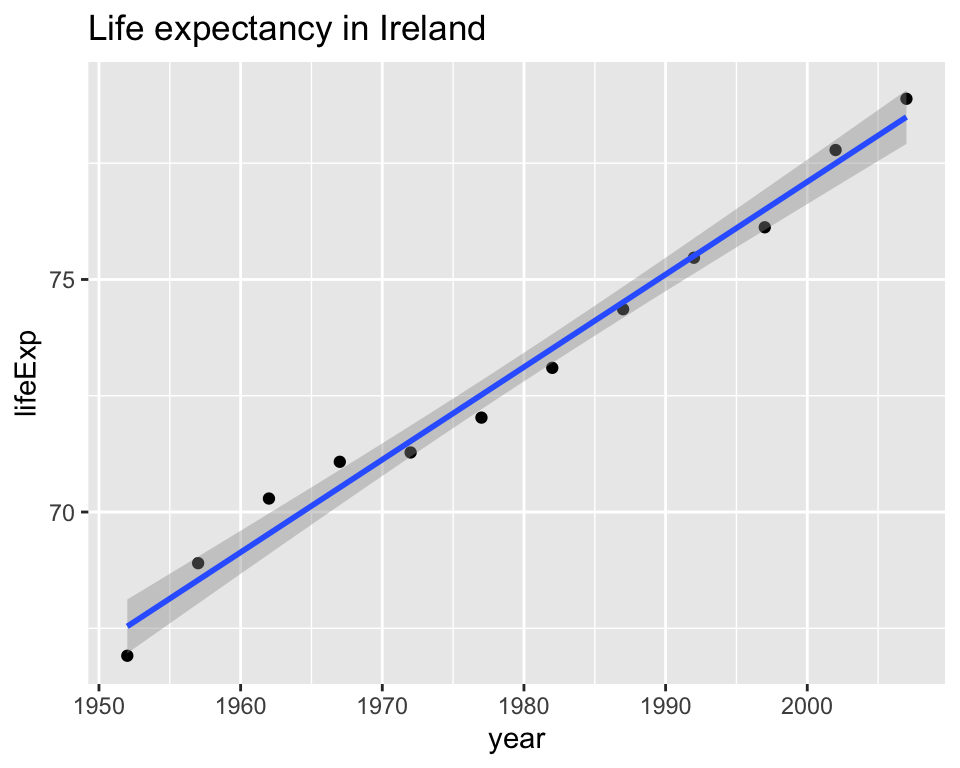We can confirm that it’s a pretty good model for other countries as well, though not for all of them

ggplot(data = gapminder, aes(x = year, y = lifeExp)) +
facet_wrap( ~ country) +
geom_point() +
geom_smooth(method = "lm")
## geom_smooth() using formula 'y ~ x'